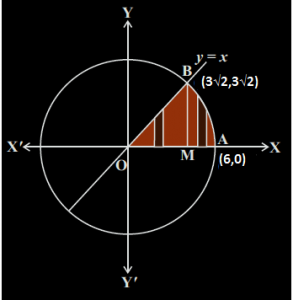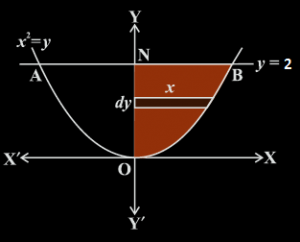# Finding Area Between A Curve And A Line

## Finding area between a curve and a line

In the previous article of Area under the curve, we discussed how to find the area enclosed by a curve and the x-axis between a given set of x-coordinates. In the upcoming discussion, we will try finding the area between a curve and a line, between a given set of points. We can divide this article in three cases i.e. when the given curve is a Circle, a parabola or an ellipse. We will be dealing with these curves in this discussion.

Let us try to understand the above concept using some examples.

Example: Find the area of the region in the first quadrant enclosed by the x-axis, the line y=x and the circle, x2+y2=36

Solution: Before finding the enclosed area, we need to solve the given equation of the curve and the line, to find their points of intersection. On solving the equations,  x2+y2=36 and y=x, we see that the points of intersection are (3√2,3√2) and (-3√2,-3√2)

After finding the point of intersection, draw a perpendicular from point B to the x-axis, meeting it at the point M.

The total shaded region is OMABO, which can be further divided into two regions i.e. OBMO and BMAB. The graph of the given line and the curve can be plotted as shown below:Let us find the areas of the regions OBMO and BMAB separately.

Area of the region OBMO is given as:

$$\begin{array}{l}\int_{0}^{3\sqrt{2}} y.dx = \int_{0}^{3\sqrt{2}} x.dx = \frac{1}{2} \left ( x^{2} \right )_{0}^{3\sqrt{2}}\end{array}$$

$$\begin{array}{l}= 9\end{array}$$

Now let us find the area of the region BMAB:

$$\begin{array}{l}\int_{3\sqrt{2}}^{6} y.dx = \int_{3\sqrt{2}}^{6} \sqrt{36 – x^{2}}.dx\end{array}$$

=

$$\begin{array}{l}\left ( \frac{1}{2}\sqrt{36-x^{2}} + \frac{1}{2} \times 36 \times \sin^{-1}\left ( \frac{x}{6} \right ) \right )_{3\sqrt{3}}^{6}\end{array}$$

= [½ x 6 x 0 + ½ x 36 x π/2] – [ ½ x 3√2 x 3√2 + ½ x 36 x π/4] = 9π – 9 – 9π/2 = 9π/2-9

Now adding the areas of the two regions we get,

9 + 9π/2-9

= 9π/2 sq.units.

Note that, in case the value of the area of some particular region comes out to be negative, we need to take the absolute value of the area and add it up to the remaining areas.

Consider another example when we have a parabola.

Example: Find the area of the region bounded by the curve y=x2 and the line y=2.

Solution: For the above case, we would get the following figure,From the given figure, we can see that the parabola is symmetric about the y-axis. So, if we find the enclosed area on one side of the y-axis and double it up, we will get the area of the complete region.  Hence, for this case, we need to consider horizontal strips, starting from y=0 to y=2.

Hence, the equation for area will be,

2 x (area of the region ONBO, bounded by the curve, y-axis and the lines y=0 and y=2)

$$\begin{array}{l}2\int_{0}^{2} x.dy = 2\int_{0}^{2}\sqrt{y} dy = 2 \times \frac{2}{3} \left ( y^{3/2} \right )_{0}^{2}\end{array}$$

=

$$\begin{array}{l}= \frac{2^{7}}{3}\end{array}$$
sq.units

In the same way, we can find the enclosed area in case of an ellipse.